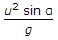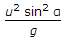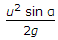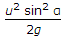# Civil Engineering - Applied Mechanics

1.
The following factor affects the orbit of a satellite up to an altitude of 720 km from the earth's surface
uneven distribution of the gravitational field
gravity of the sun and the moon
aerodynamic forces
none of these.
Explanation:
No answer description is available. Let's discuss.

2.
If α and u are the angle of projection and initial velocity of a projectile respectively, the horizontal range of the projectile, isExplanation:
No answer description is available. Let's discuss.

3.
Newton's law of Collision of elastic bodies states that when two moving bodies collide each other, their velocity of separation
is directly proportional to their velocity of approach
is inversely proportional to their velocity of approach
bears a constant ratio to their velocity of approach
is equal to the sum of their velocities of approach.
Explanation:
No answer description is available. Let's discuss.

4.
Two forces of 6 Newtons and 8 Newtons which are acting at right angles to each other, will have a resultant of
5 Newtons
8 Newtons
10 Newtons
12 Newtons.